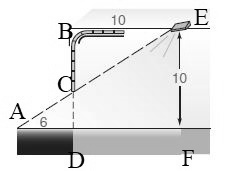Chapter 5.2, Problem 32EElementary Geometry For College St...

7th Edition
Alexander + 2 others
ISBN: 9781337614085

Solutions

Chapter
SectionElementary Geometry For College St...

7th Edition
Alexander + 2 others
ISBN: 9781337614085
Textbook Problem

In Exercise 29 to 32, use the fact that triangles are similar.As a garage door closes, light is case 6 ft beyond the base of the door (as shown in the accompanying drawing) by a light fixture that is set in the garage ceiling 10 ft back from the door. If the ceiling of the garage is 10 ft above the floor, how far is the garage door above the floor at the time that light is cast 6 ft beyond the door?To determine

To calculate:

The distance when the garage door above the floor at the time that light is cast 6ft beyond the door.

Explanation

Given:

As a garage door closes, light is cast 6ft beyond the base of the door by a light fixture that is set in the garage ceiling 10ft back from the door. If the ceiling of the garage is 10ft above the floor.

Definition Used:

Similarity between Two polygons:

Two polygons are similar if and only if when all pairs of corresponding angles are congruent and all pairs of corresponding sides are proportional.

Calculation:

According to the segment-addition postulate, BD=BC+CD.

Let us assume that CD=x and BC=10x.

According to the definition of similar polygons, CDBC=ADBF

Still sussing out bartleby?

Check out a sample textbook solution.

See a sample solution

The Solution to Your Study Problems

Bartleby provides explanations to thousands of textbook problems written by our experts, many with advanced degrees!

Get Started

Add the following fractions and reduce to lowest terms. 13+45

Contemporary Mathematics for Business & Consumers

Proof prove that cos(sin1x)=1x2

Calculus: Early Transcendental Functions (MindTap Course List)

True or False: Let . Then f(x, y) is a joint probability function.

Study Guide for Stewart's Multivariable Calculus, 8th

∫(x − cos x)dx = 1 − sin x + C 1 + sin x + C

Study Guide for Stewart's Single Variable Calculus: Early Transcendentals, 8th